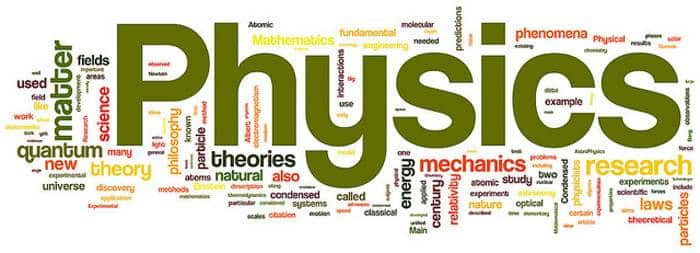# Plane Mirror and Spherical Mirror Question / Answers### Plane Mirror and Spherical Mirror Online Question Bank:

Ques: What should be the angle between two plane mirrors so that whatever be the angle of incidence, the incident ray and the reflected ray from the two mirrors be parallel to each other
(a) 60o
(b) 90o
(c) 120o
(d) 175o
Ans. (b)

Ques: A light bulb is placed between two plane mirrors inclined at an angle of 60o. The number of images formed are
(a) 6
(b) 2
(c) 5
(d) 4
Ans. (c)

Ques: A man is 180 cm tall and his eyes are 10 cm below the top of his head. In order to see his entire height right from toe to head, he uses a plane mirror kept at a distance of 1 m from him. The minimum length of the plane mirror required is
(a) 180 cm
(b) 90 cm
(c) 85 cm
(d) 170 cm
Ans. (b)

Ques: A small object is placed 10 cm infront of a plane mirror. If you stand behind the object 30 cm from the mirror and look at its image, the distance focused for your eye will be
(a) 60 cm
(b) 20 cm
(c) 40 cm
(d) 80 cm
Ans. (c)

Ques: A ray is reflected in turn by three plain mirrors mutually at right angles to each other. The angle between the incident and the reflected rays is
(a) 90o
(b) 60o
(c) 180o
(d) None of these
Ans. (c)

Ques: Two plane mirrors are inclined at an angle of 72o. The number of images of a point object placed between them will be
(a) 2
(b) 3
(c) 4
(d) 5
Ans. (c)

Ques: A watch shows time as 3 : 25 when seen through a mirror, time appeared will be
(a) 8 : 35
(b) 9 : 35
(c) 7 : 35
(d) 8 : 25
Ans. (a)

Ques: A man of length h requires a mirror, to see his own complete image of length at least equal to
(a) h/4
(b) h/3
(c) h/2
(d) h
Ans. (c)

Ques: Focal length of a plane mirror is
(a) Zero
(b) Infinite
(c) Very less
(d) Indefinite
Ans. (b)

Ques: Given a point source of light, which of the following can produce a parallel beam of light
(a) Convex mirror
(b) Concave mirror
(c) Concave lens
(d) Two plane mirrors inclined at an angle of 90o
Ans. (b)

Related: Quiz: Endocrine System

Ques: A virtual image three times the size of the object is obtained with a concave mirror of radius of curvature 36 cm. The distance of the object from the mirror is
(a) 5 cm
(b) 12 cm
(c) 10 cm
(d) 20 cm
Ans. (b)

Ques: A diminished virtual image can be formed only in
(a) Plane mirror
(b) A concave mirror
(c) A convex mirror
(d) Concave-parabolic mirror
Ans. (c)

Ques: While using an electric bulb, the reflection for street lighting should be from
(a) Concave mirror
(b) Convex mirror
(c) Cylindrical mirror
(d) Parabolic mirror
Ans. (b)

Ques: The field of view is maximum for
(a) Plane mirror
(b) Concave mirror
(c) Convex mirror
(d) Cylindrical mirror
Ans. (c)

Ques: A person sees his virtual image by holding a mirror very close to the face. When he moves the mirror away from his face, the image becomes inverted. What type of mirror he is using?
(a) Plane mirror
(b) Convex mirror
(c) Concave mirror
(d) None of these
Ans. (c)

Ques: If an object is placed 10 cm infront of a concave mirror of focal length 20 cm, the image will be
(a) Diminished, upright, virtual
(b) Enlarged, upright, virtual
(c) Diminished, inverted, real
(d) Enlarged, upright, real
Ans. (b)

Ques: The minimum distance between the object and its real image for concave mirror is
(a) f
(b) 2f
(c) 4f
(d) Zero
Ans. (d)

Ques: The focal length of a convex mirror is 20 cm its radius of curvature will be
(a) 10 cm
(b) 20 cm
(c) 30 cm
(d) 40 cm
Ans. (d)

Ques: A point object is placed at a distance of 30 cm from a convex mirror of focal length 30cm. The image will form at
(a) Infinity
(b) Focus
(c) Pole
(d) 15 cm behind the mirror
Ans. (d)

Ques: For a real object, which of the following can produced a real image
(a) Plane mirror
(b) Concave lens
(c) Convex mirror
(d) Concave mirror
Ans. (d)

Ques: Convergence of concave mirror can be decreased by dipping in
(a) Water
(b) Oil
(c) Both
(d) None of these
Ans. (d)

### About the author

#### Richa Bhardwaj

Richa (B. Tech) has keen interest in Science and loves to teach students about it through lectures and assignments. She always try to use simple language and sentences while writing to make sure learner understands everything properly.

### 1 Comment

• aditya raj says:

great questions!